# Understand How to Effectively Monitor Signal Voltage and Power with RMS-to-DC Converters

By Art Pini

Contributed By Digi-Key's North American Editors

Switched mode power conversion and industrial controllers use switch mode transistor, semiconductor controlled rectifiers, and related thyristor devices to control power by adjusting the duty cycle of the input waveforms. The waveforms produced are very complex, so to measure and monitor their power levels, designers must determine the root mean square (RMS) level of current and voltage waveforms. This is where RMS-to-DC converters can help.

While it’s possible to perform programmed calculations on a waveform acquired by an oscilloscope to determine the RMS value, this can be time consuming. RMS-to-DC converters simplify power measurements by outputting a DC level proportional to the input waveform RMS level in real-time. They are employed widely in power monitoring controls and instruments to measure the RMS level of complex, non-sinusoidal waveforms.

This article will explain RMS and power computations concepts. It will also describe how RMS-to-DC converters work and how they are to be applied.

## Measuring complex waveforms

Complex waveforms can be difficult to deal with as modern electronics no longer use just DC or sinusoidal voltage waveforms (Figure 1). How do you quantify them?  What measurements really describe any of these waveforms?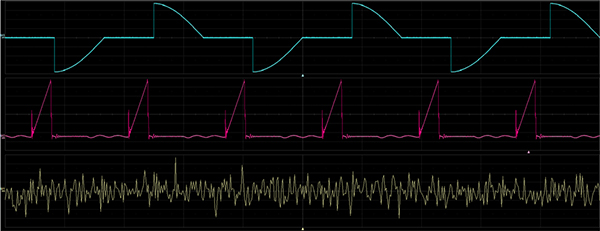Figure 1: Commonly encountered complex waveforms; thyristor based AC controller (top), current in a switched mode power supply (center), random Gaussian noise (bottom). (Image source: Digi-Key Electronics)

The top waveform is from a thyristor-based AC controller. It has a zero average value and its peak-to-peak amplitude does not relate linearly to its power, especially at low duty cycles. The center waveform is the current through a power FET in a switched mode power supply. The bottom waveform is broadband noise. It is a non-periodic waveform that also has a zero average (mean) value and can have a very high peak value, but it has finite average power.

Early AC voltmeters used full-wave rectified average readings to measure the effective value of the voltage. These voltmeters worked fine for sinewaves, but complex waveforms produced incorrect readings. The only technique that produces waveform independent effective values is the RMS measurement.

## What is RMS?

RMS measurement is broadly recognized to furnish the most accurate amplitude information about a waveform. It is a coherent, impartial, and standard way to measure and compare dynamic signals, regardless of the wave shape.

The RMS is a fundamental measurement of the magnitude of an AC signal. The RMS value assigned to the signal is the DC level required to produce an equal amount of heating in the same load. As such, it is related to the signal power.

The mathematical definition of the RMS value of a waveform is defined as the value obtained by squaring the signal, taking the average, and then taking the square root. The averaging time window must be of suitable length to allow filtering at the lowest frequencies required of the measurement. In equation form, the RMS value of a waveform over time is: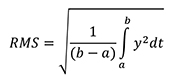The RMS value is the square root of the mean squared voltage. The mean squared voltage divided by the load impedance is the average power delivered by the waveform, which again shows that RMS is related to the signal power.

The equation can be applied numerically to a waveform acquired by an instrument like an oscilloscope. Numerical calculation requires a fair amount of program coding. Measuring a physical waveform without having to digitize it is the very useful function of an RMS-to-DC converter.

## RMS-to-DC converter

As its name implies, the RMS-to-DC converter is a device that produces a DC output level proportional to the RMS amplitude of an input signal. Historically, the first such devices were instruments that actually measured heat produced by the input waveform that was connected to a load. These have long been supplanted by integrated circuits that perform the same task electronically.

There are three possible methods to compute the RMS amplitude of a waveform: explicit, implicit, and delta-sigma circuit topologies (Figure 2).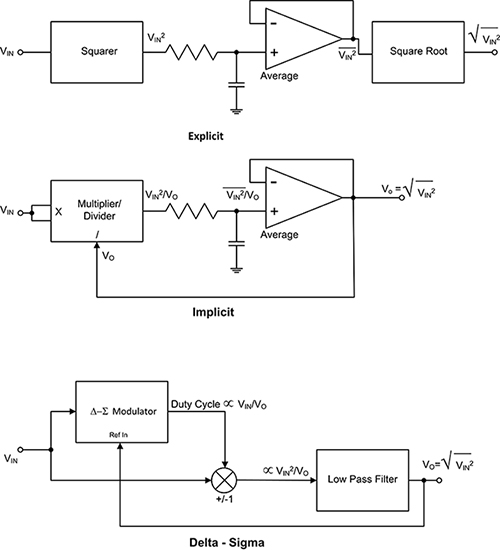Figure 2: There are three different ways of measuring the RMS value of a waveform: explicit, implicit, and delta-sigma circuit topologies. (Image source: Digi-Key Electronics)

With the explicit method, the signal is squared, averaged, and then the square root is extracted. The square and square root extraction is generally implemented using log-antilog transistor arrays. Averaging is accomplished using an RC low pass filter, usually requiring an external capacitor to set the cutoff frequency. This method works, but the square root operation results in measurements that have a very high dynamic range which raises the possibility of very large errors.

The second method is called the implicit method. It improves the performance of the explicit method by rearranging the mathematical operations using feedback. The input stage is a multiplier/divider with the output being fed back as the divisor. This is a clever approach to avoiding the square root operation, as shown in equations 2 through 5: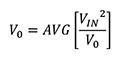Because VO is a DC level its value is equal to its average value: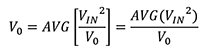Multiplying both side of the equation by VO: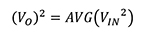Finally, taking the square root of both sides of the equation: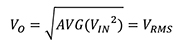The AD737JRZ-RL from Analog Devices is an RMS-to-DC converter that uses the implicit computation technique. It has an accuracy of ±0.2 mV ±0.3% of reading. In addition to outputting the RMS value of the input signal, it also provides the averaged rectified value and the absolute value.

The final technique for determining RMS is the delta-sigma method. In this method a delta-sigma (ΔΣ) modulator is used as the divider. A simple polarity switch at the output of the modulator serves as a multiplier. The output of the ΔΣ is a pulse with an average duty cycle proportional to the ratio of the input signal to the output signal. This output pulse drives the polarity switch between gain values of +1 and -1, resulting in the output being proportional to the ratio of the input squared to the output. The low pass filter provides averaging. The same math used for the implicit method applies in the ΔΣ technique, resulting in an output equal to the RMS value of the input signal. One of the advantages of this method is that the computation is faster, resulting in a higher measurement bandwidth.

The Analog Devices LTC1966IMS8#TRPBF is an RMS-to-DC converter that uses the ΔΣ method. It has a bandwidth of 800 kHz with less than 0.25% total error for input signals under 1 kHz. Due to the excellent linearity of the technique, the measurement linearity is 0.02%.

## Applying RMS-to-DC converters

RMS-to-DC converters are used whenever it is necessary to monitor or control signal levels. These can be complex waveforms or more traditional sine waves. Consider a three-phase power monitoring application (Figure 3).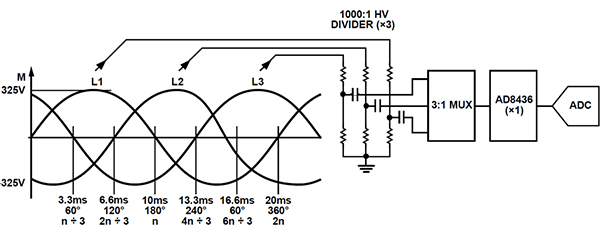Figure 3: Using an RMS-to-DC converter to monitor a 50 Hz, three-phase power line. (Image source: Analog Devices)

In this application, the Analog Devices AD8436 RMS-to-DC converter is used along with a 3:1 multiplexer (mux), allowing a single RMS-to-DC converter to monitor the three phases. The phase voltages are sampled using three 1000:1 high-voltage dividers. The output of the RMS-to-DC converter is routed to an analog-to-digital converter (ADC). The mux and ADC continuously sample all phases well within the period of a single 20 ms power line voltage period.

The AD8436 is a low-power RMS-to-DC converter using implicit topology. Its accuracy is ±10 microvolts (µV) ± 0.25% with a 1 MHz bandwidth. It features a built-in FET buffer to interface with external attenuators. It also has an output buffer amplifier that minimizes errors when driving low-impedance loads.

## Measuring non-periodic waveforms

RMS-to-DC converters can also be used to characterize non-periodic signals like Gaussian noise (Figure 4).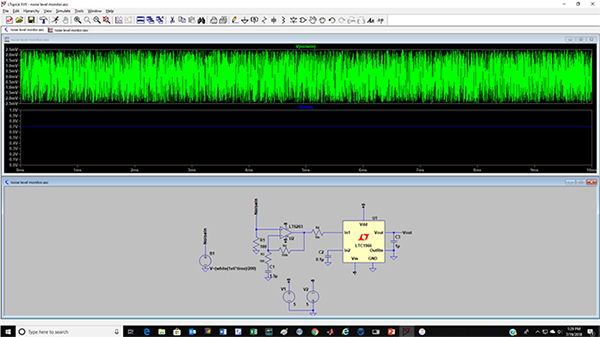Figure 4: An LTSpice XVII simulation of a noise level monitor circuit, recommended by the manufacturer, using an Analog Devices LTC1966 RMS-to-DC converter. (Image source: Digi-Key Electronics)

Noise and noise-like signals are very hard to characterize. Gaussian noise, for instance, can have very high peak-to-peak amplitude (theoretically infinite). The peak-to-peak levels are essentially unbounded and grow with increased observation time. The RMS level however, is finite and very well behaved. The noise monitor circuit, modeled in LTSpice XVII, uses an Analog Devices LTC1966 ΔΣ RMS-to-DC converter. The operational amplifier ahead of the LTC1966 boosts the noise amplitude by a gain factor of 1000. The 1 microfarad (µF) capacitor at the output is the averaging capacitance that sets the corner frequency of the average filter. The output of the RMS-to-DC converter is a DC level with a sensitivity of approximately 1 millivolt (mV) DC per mV RMS of noise. In this example it reads 0.7 volts, indicating a noise amplitude of 700 mVRMS.

In a similar way, the RMS level of the switched mode power supply current waveform can be measured (Figure 5).

In this LTSpice XVII simulation, the actual waveform has been imported into the piece-wise linear (PWL) current source. The current is sensed using a one ohm resistive shunt so that the input voltage to the LTC1966 is mapped as 1 mV/mA. This signal does not require the amplifier used previously, and the current is sensed directly by the LTC1966. The peak current of the waveform is 0.584 A. The ramp waveform has a duty cycle of 20%. The measured RMS voltage at the RMS-to-DC converter output is 140 mV, translating to an RMS current amplitude of 140 mA.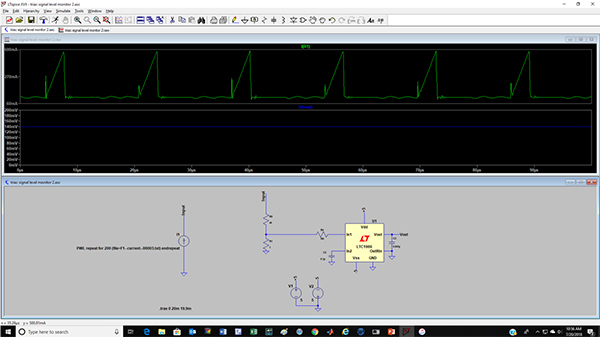Figure 5: A simulation of the LTC1966 used to measure the RMS value of a switching FET current waveform of a switched mode power supply. (Image source: Digi-Key Electronics)

The thyristor-based controller waveform can similarly be measured in simulation (Figure 6).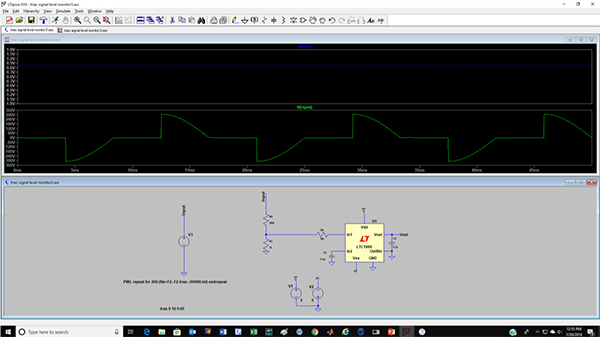Figure 6: The thyristor-based controller waveform, simulated and measured using the LTC1966, has an RMS value of 155 volts. (Image source: Digi-Key Electronics)

Again, the actual waveform was imported and used as a PWL source. The 620 volt peak-to-peak waveform is attenuated with a 200:1 voltage divider. The resulting RMS output of the LTC1966 RMS-to-DC converter is 0.767 volts, represented in RMS level at the circuit input of 155 volts.

## Conclusion

The use of analog RMS-to-DC converters simplifies the measurement of the effective power of the most complex signals without requiring writing and debugging a lot of programming code. These low-cost converters are ideal for measurement, or for monitoring and control of power-related parameters of various waveforms.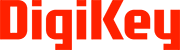Disclaimer: The opinions, beliefs, and viewpoints expressed by the various authors and/or forum participants on this website do not necessarily reflect the opinions, beliefs, and viewpoints of Digi-Key Electronics or official policies of Digi-Key Electronics.